## Real line, decimals and significant figures(实线，小数，和有效数字)

### Sets and elements

A set is a collection of objects, referred to as elements. A set may be represented, for example, by a list of elements surrounded by curly brackets and separated by commas, or using set builder notation {…|…}, where the vertical line is an abbreviation for “such that”. For example, { x | x is a natural number less than 5 }
and {0, 1, 2, 3, 4} represent the same set, whose elements are precisely 0, 1, 2, 3 and 4.
(A natural number is a whole counting number, including zero. Note that some people do not count zero as a counting number, but we do in this course!)

### Element symbol

The symbol $\in$ is an abbreviation for “is an element of”, and $\notin$ is an
abbreviation for “is not an element of”. For example, if

$A=\{x | x \text { is a natural number less than } 5\}$

then $2 \in A$, but $5 \notin A$.

### Subset symbols

If A and B are sets and we write $A \subseteq B$ or $B \supseteq A$, then we mean that every element of A is also an element of B, and say that A is a subset of B. For example
$\{1,2,3\} \subseteq\{1,2,3,4\}$ and $\{1,2,3,4\} \supseteq\{1,2,3\}$, but $\{1,2,3,4\} \nsubseteq\{1,2,3\}$.

### Equality of sets

If A and B are sets then A = B if and only if $A \subseteq B$ and $B \subseteq A$, that is, A and B have precisely the same elements. Order and repetition are not important.
For example, {1, 2, 3, 4} = {4, 1, 3, 2} = {4, 1, 3, 1, 2, 3}.

### Intersection, union and slash

If A and B are sets then put

• $A \cap B=\{x | x \in A \text { and } x \in B\}$, called the intersection of A and B.
• $A \cup B=\{x | x \in A \text { or } x \in B\}$, called the union of A and B.
• $A \backslash B=\{x | x \in A \text { and } x \notin B\}$, called A slash B, the result of removing from A all elements from B.

### Natural numbers

The set $\mathbb{N}=\{0,1,2,3, \ldots\}$ of natural numbers forms a number system, closed under addition and multiplication, by which we mean that if m and n are natural numbers, then m+n and mn (the result of multiplying m by n) are also natural numbers.

### Integers

The set $\mathbb{Z}=\{0, \pm 1, \pm 2, \pm 3, \ldots\}$ of integers forms a number system, closed under addition, subtraction and multiplication.

### Rationals

The set $\mathbb{Q}=\{a / b | a, b \in \mathbb{Z}, b \neq 0\}$ of fractions, also called rational numbers (derived from the word ratio), forms a number system, closed under addition, subtraction, multiplication and division by nonzero elements. To add and multiply rational numbers, use the rules

$\frac{a}{b}+\frac{c}{d}=\frac{a d+b c}{b d} \quad \text { and } \quad \frac{a}{b} \cdot \frac{c}{d}=\frac{a c}{b d}$

### Decimal expansion of a real number

A nonnegative real number $\alpha$ has a decimal expansion

$\alpha=b_{n} b_{n-1} \ldots b_{2} b_{1} \cdot a_{1} a_{2} a_{3} \ldots$

where the $b_{i}$ and $a_{j}$ are digits from the set {0, 1, 2, . . . , 9}.

### Recurring decimal expansions

A real number is rational if and only if it has a recurring
decimal expansion, which means the pattern of digits repeats forever from some point
onwards. For example,

$\frac{6}{7}=0.857142857142 \ldots=0 . \dot{8} 5714\dot{2} ,$

where the two dots indicate the start and finish of the pattern of digits that gets repeated.

### The real number line

The real numbers form a number system $\mathbb{R}$ that is closed under addition, subtraction, multiplication and division by nonzero elements. We visualise $\mathbb{R}$ as a continuous number line, called the real line, with zero in the middle, negative numbers to the left and positive numbers to the right.

### The number $\pi$

The real number $\pi$ = 3.14159… is the ratio of the perimeter of a circle to its diameter. The fact that $\pi$ is the same for all circles is an advanced argument involving limits (a concept explained in Module 3) that relies on the fact that circles can be approximated arbitrarily well using similar triangles and the fact that ratios of corresponding sides of similar triangles are equal.

### Irrationals

A real number that is not rational (that is, cannot be expressed as a fraction
involving a $\sqrt{2}$ , $\sqrt{3}$ and $\pi$ are irrational, though the proof for $\pi$ is difficult. (A proof that $\sqrt{2}$ is irrational is given in the notes
accompanying Module 1, Video 1.2.)

### Significant figures

Real numbers may be approximated by rational numbers with finite decimal expansions. The number of digits counted to the right from the leftmost positive digit is called the number of significant figures. For example, real numbers represented by 26.103, 0.00304 and 0.003040 are quoted to 5, 3 and 4 significant figures respectively.

### Scientific notation

A positive real number $\alpha$ is expressed in scientific notation if it has the form

$\alpha=b \cdot a_{1} a_{2} \ldots a_{m} \times 10^{k}$

where m is nonnegative and k is an integer. For example, 193.034 and 0.003040 become $1.93034 \times 10^{2}$ and $3.040 \times 10^{-3}$ respectively in scientific notation. The number of digits used in scientific notation is the number of significant figures being quoted, and this avoids ambiguity in the case of large whole numbers (with zeros as place-holders).

### Accuracy rule for addition and subtraction

When adding or subtracting numbers, the final answer should be quoted to the least number of decimal places that occurs.

### Accuracy rule for multiplication and division

When multiplying or dividing numbers, the final answer should be quoted to the least number of significant figures that occurs.

## The Theorem of Pythagoras and properties of the square root of 2(毕达哥拉斯定理和2的平方根性质)

### Square roots

If $\alpha \in \mathbb{R}$ then $\sqrt{\alpha}=\beta$ if and only if $\beta \geq 0$ and $\beta^{2}=\alpha$. In particular, $\sqrt{0}=0$.
If $\sqrt{\alpha}>0$ then we call $\sqrt{\alpha}$ the positive square root and $-\sqrt{\alpha}$ the negative square root of $\alpha$.
For example, 2 is the positive square root of 4, and -2 is the negative squareroot of 4.
We also write $\alpha^{\frac{1}{2}}$ for $\sqrt{\alpha}$, and note the following laws hold, where $\alpha,\beta \geq 0$ and $\gamma>0$:

$\alpha^{\frac{1}{2}} \beta^{\frac{1}{2}}=\sqrt{\alpha} \sqrt{\beta}=\sqrt{\alpha \beta}=(\alpha \beta)^{\frac{1}{2}}$

and

$\frac{\alpha^{\frac{1}{2}}}{\gamma^{\frac{1}{2}}}=\frac{\sqrt{\alpha}}{\sqrt{\gamma}}=\sqrt{\frac{\alpha}{\gamma}}=\left(\frac{\alpha}{\gamma}\right)^{\frac{1}{2}}$

Negative real numbers do not have square roots within the real number system $\mathbb{R}$ (though they do have square roots in the larger complex number system $\mathbb{C}$).

### Surds

Any expression involving a square root symbol √ is called a surd expression.
For example,

$\sqrt{2}, \quad 2 \sqrt{3}, \quad \sqrt{2}+\sqrt{3}, \quad 4-\sqrt{2}, \quad \frac{1}{2+\sqrt{5}}, \quad \sqrt{a^{2}+b^{2}}, \quad \frac{\sqrt{a+\sqrt{b+2}}}{2 \sqrt{c}}$

are all surd expressions. (More generally, surd expressions can involve other types of roots, such as cube roots, or tenth roots, but simple square roots are the most common.)

### The Distributive Law (expanding brackets)

If $a, b, c \in \mathbb{R}$ then

$(a+b) c=a c+b c \quad \text { and } \quad a(b+c)=a b+a c$

It follows that, if $a, b, c, d \in \mathbb{R}$, then

$(a+b)(c+d)=a c+a d+b c+b d$

### Squares and differences of squares

As a consequence of distributivity, we have the following identities, for all $a, b \in \mathbb{R}$:

$(a+b)^{2}=a^{2}+2 a b+b^{2}, \quad(a-b)^{2}=a^{2}-2 a b+b^{2}, \quad a^{2}-b^{2}=(a+b)(a-b)$

The last identity is called the difference of two squares formula, and it features in many
algebraic tricks or manipulations. For example,

$77 \times 83=(80-3)(80+3)=80^{2}-3^{2}=6400-9=6391$

and, for any positive reals a and b,

$(\sqrt{a}+\sqrt{b})(\sqrt{a}-\sqrt{b})=a-b$

### Theorem of Pythagoras

If a and b are the lengths of the shorter sides of a right angled triangle, and c is the length of the hypotenuse (the longest side), then

$a^{2}+b^{2}=c^{2}$

so that $c=\sqrt{a^{2}+b^{2}}, a=\sqrt{c^{2}-b^{2}}$ and $b=\sqrt{c^{2}-a^{2}}$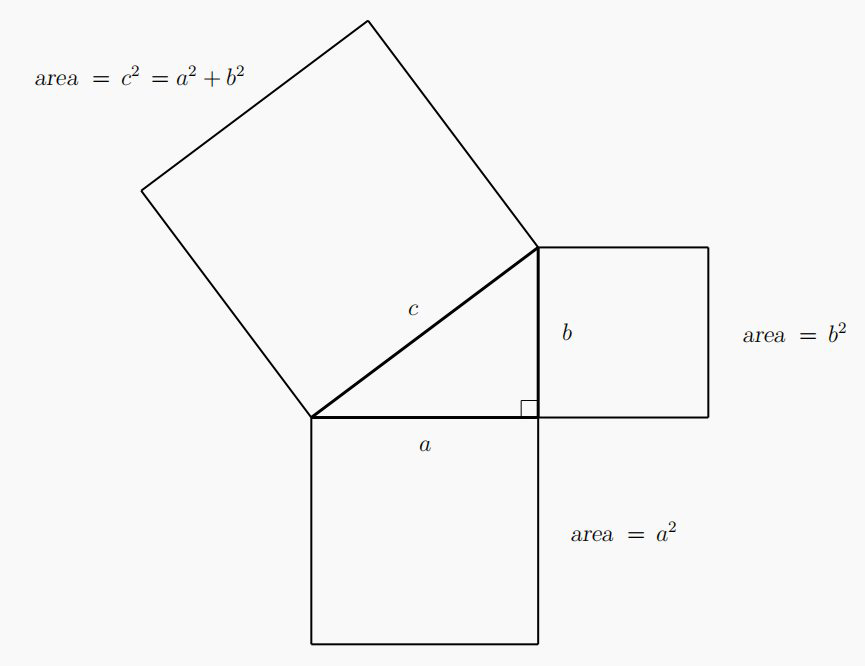### Irrational surds

If $n \in \mathbb{N}$ then $\sqrt{n}$ is rational if and only if $n$ is a perfect square, that is $n=m^{2}$ for some $m \in \mathbb{N} .$ Thus, for example, $\sqrt{2}, \sqrt{3}, \sqrt{5}$ and $\sqrt{6}$ are irrational.

## Algebraic expressions, surds and approximations

### Differences of two squares formula

In any well-behaved arithmetic we have the identity

$a^{2}-b^{2}=(a+b)(a-b)$

known as the difference of two squares formula. It is exploited, for example, in the next important idea (rationalising the denominator).

### Rationalising the denominator

If $a \in \mathbb{Z}, b \in \mathbb{N}$ and $a \pm \sqrt{b} \neq 0$ then

$\frac{1}{a \pm \sqrt{b}}=\left(\frac{1}{a \pm \sqrt{b}}\right)\left(\frac{a \mp \sqrt{b}}{a \mp \sqrt{b}}\right)=\frac{a \mp \sqrt{b}}{a^{2}-b} .$

For example,

$\frac{1}{1+\sqrt{5}}=\left(\frac{1}{1+\sqrt{5}}\right)\left(\frac{1-\sqrt{5}}{1-\sqrt{5}}\right)=\frac{1-\sqrt{5}}{1-5}=\frac{\sqrt{5}-1}{4},$

and

$\frac{1}{3-\sqrt{7}}=\left(\frac{1}{3-\sqrt{7}}\right)\left(\frac{3+\sqrt{7}}{3+\sqrt{7}}\right)=\frac{3+\sqrt{7}}{9-7}=\frac{3+\sqrt{7}}{2} .$

It is a fact, discussed below, that if $a \in \mathbb{Z}$ and $b \in \mathbb{N},$ such that $b$ is not a perfect square, then $a \pm \sqrt{b}$ is irrational. The terminology rationalising the denominator comes about, as in the examples above, where the original fraction has an irrational number in the denominator. The fraction is then transformed by this technique, so that the denominator becomes rational (an integer, in fact, in each of these examples).

### Continued fractions

An expression obtained by taking a number and adding a reciprocal, where the denominator itself is another expression involving a number added to a reciprocal, and allowing this process to repeat, is called a continued fraction.
If the entire expression is just a reciprocal, but the denominator can be expressed symbolically in terms of the entire expression, then the expression can be fed into itself indefinitely, to obtain an infire continued fraction. For example, the expression

$X=\frac{1}{1+X}$

leads to the infinite continued fraction

$X=\frac{1}{1+\frac{1}{1+\frac{1}{1+\frac{1}{1+...}}}}$

By truncating such an expression at some place, one obtains a finite continued fraction, which then evaluates to an ordinary fraction.

## Equations and inequalities

### Positive, negative, nonnegative and nonpositive real numbers

A real number $x$ is called positive if it appears to the right of zero on the real number line, in which case we write $a>0,$ and negative if it appears to the left of zero, in which case write $a<0 .$ We say that $x$ is nonnegative if it is positive or zero, in which case we write $a \geq 0,$ and nonpositive if it is negative or zero, in which case we write $a \leq 0 .$

### Multiplication and (signed) areas of rectangles

If $a$ and $b$ are real numbers then the product of $a$ and $b$ is the result of multiplying $a$ by $b$ , denoted typically by $a \times b$ or $a b$ (juxtaposition). The product $a b$ may be represented, geometrically, by the (signed) area of a rectangle with side lengths $a$ and $b$ . If both $a$ and $b$ are nonnegative then this is just the usuale nonnegative) area.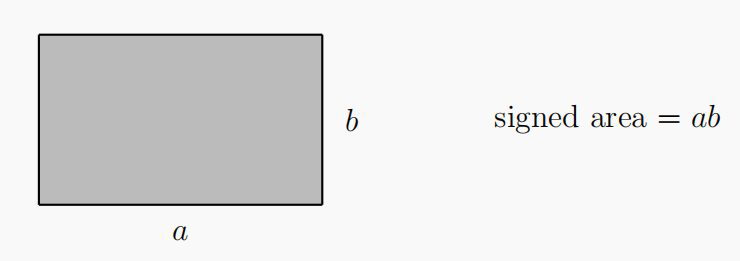If one of $a$ and $b$ is positive and the other negative then the area $a b$ of the rectangle is regarded as negative.
If both $a$ and $b$ are negative then the area $a b$ is regarded as positive.
The formal rigorous explanation for this last fact is based on laws, or axioms, of arithmetic, and involves an advanced argument in abstract algebra that one typically sees in second or third year university mathematics.

Intuitively, one can just think of negatives of negatives being positive. It wouldn’t make sense for the negative of a negative number to remain negative. This is mirrored in common speech when one uses double negatives: for example, to say “I didn’t not say that!” is a complicated way of saying “I said that!” (though its use can help to emphasise the positive in a heated argument.) (The speech analogy also can break down, as some languages, including some colloquial English, use a double negative in certain turns of phrase to reinforce a single negative.)
For example, 2 × 2 = 4 and

$2 \times(-2)=(-2) \times 2=-4$

but

$(-2) \times(-2)=-(2 \times(-2))=-(-4)=4$

### Factorisation of zero

If a and b are real numbers then the product ab is zero if and only if a = 0 or b = 0 (including the possibility that a = b = 0).
This follows from the representation of the product ab as the (signed) area of a rectangle with side length a and b. If the area vanishes, then at least one of the side lengths must vanish.
This fact about the factorisation of zero is very useful for solving equations. For example,
if x is a real number such that

$(x-1)(x-2)=0$

then either x - 1 = 0 or x - 2 = 0, so that either x = 1 or x = 2.
This fact clearly generalises to more than two factors. For example, if

$(x-4)(x+1)(x+3)=0$

then either x - 4 = 0, x + 1 = 0 or x + 3 = 0, so that either x = 4, x = -1 or x = -3.

### Inequalities

Let $a, b \in \mathbb{R} .$ We write $a if $a$ appears to the left of $b$ on the real number line, which is equivalent to the difference $b-a$ being positive (and the difference $a-b$ being negative).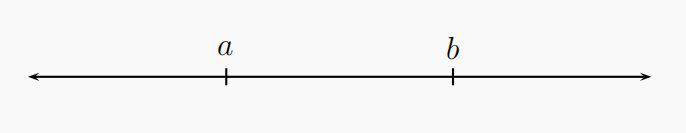In this case we say that $a$ is less than $b$ and $b$ is greater than $a,$ and also write $b>a .$
We write $a \leq b$ if $a=b$ or $a and write $a \geq b$ if $a=b$ or $a>b$ .
For example,

$-10<-5<-0.1<-0.01<0<0.5<1<5$

Note that -10 < 5 even though you might think of -10 as a “larger” number than 5 in terms of “size”.
(Later, we introduce the magnitude or absolute value of a real number, which captures the idea of “size” precisely. The word “larger” is ambiguous, and has to be read in context in mathematics.)

### Properties of inequalities

Let $a, b, c \in \mathbb{R}$

• If $a and $c \leq d$ then $a+c so that, in particular, $a+c
• It follows also, from the previous part, that if $a then $a-c .
• If $a and $c>0$ then $a c and $\frac{a}{c}<\frac{b}{c}$
• If $a and $c<0$ then $a c>b c$ and $\frac{a}{c}>\frac{b}{c},$ so that, in particular, $-a>-b$ .
• If $0 then $\frac{1}{a}>\frac{1}{b}>0$ .

Variations of these properties hold using $>, \leq$ and $\geq$ .

## Sign diagrams, solution sets and intervals

### The sign of a real number

The sign of a real number x refers to whether x is positive or x is negative. We say that two numbers have the same sign if they are both positive or they are both negative. We say that two numbers have different signs if one is positive and the other is negative.
Consider real numbers a and b. The product ab is positive if and only if a and b have the same sign. The product ab is negative if and only if a and b have different signs.

### Sign diagrams

A sign diagram is a table with two rows, the first row indicating values of
some variable, x say, and the second row indicating the sign (indicated by a plus sign + for positive and a minus sign - for negative) of some particular expression involving x. The line in the table between the rows represents the real line, and important points for x are marked off, typically corresponding to x for which the expression evaluates to zero (and this is usually obvious when the expression has been factorised).

For example, here is the sign diagram for the expression $(x-1)(x-2),$ which is zero, when $x=1$ and $x=2,$ positive when $x<1$ and when $x>2,$ and negative when $1 :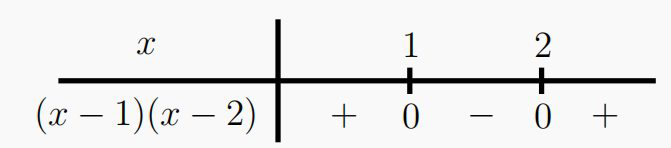Here is the sign diagram for the expression $x\left(x^{2}-1\right)=x(x+1)(x-1),$ which is zero when $x=0, x=1$ and $x=-1,$ positive when $-1 and when $x>1,$ and negative when $x<-1$ and when $0 :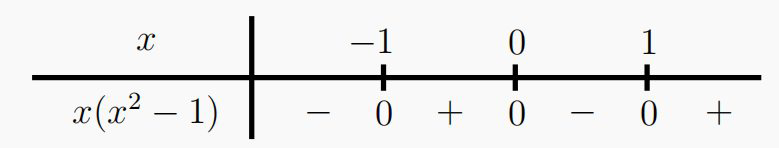One can also construct sign diagrams when an expression becomes undefined, especially
when fractions are involved where the variable appears in the denominator, in which case the letter “u” is used in the second row, as an abbreviation for “undefined”.

Here is the sign diagram for the expression $\frac{x^{2}-1}{x}=\frac{(x+1)(x-1)}{x},$ which is identical to the previous example, except for noting that the expression is undefined for $x=0$ :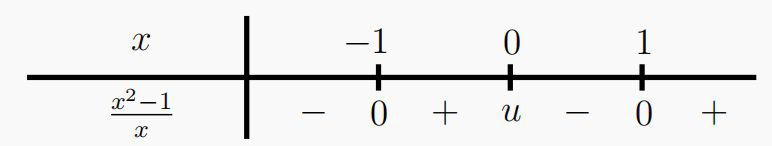For another variation, here is the sign diagram for the expression $\frac{x}{x^{2}-1}=\frac{x}{(x+1)(x-1)},$ where now the expression is undefined for $x=-1$ and $x=1 :$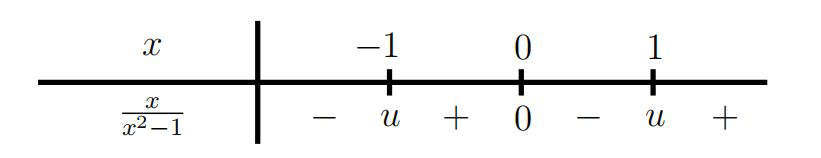### Empty set

The empty set is the set without any elements and denoted by $\emptyset .$ If $A$ is any set then $A \cup \emptyset =A$, $A \cap \emptyset = \emptyset$ and $A \backslash A=\emptyset$ .

### Solution sets

The solution set (within the real number system $\mathbb{R}$) of an equation or an inequality involving a variable x is the set of all real numbers x that satisfy the given equation or inequality. If no real numbers satisfy the given equation or inequality then the solution set is empty.
For example, the solution set of the equation 2x + 1 = 7 is the set {3}, since x = 3 is the only solution.
The solution set of the equation (x - 1)(x - 2) = 0 is the set {1, 2}, since the solutions are precisely x = 1 and x = 2.
The solution set of the inequality $2 x+1<7$ is the set $\{x \in \mathbb{R} | x<3\}$
The solution set of the inequality $2 x+1 \geq 7$ is the set $\{x \in \mathbb{R} | x \geq 3\}$
The solution set of the inequality $(x-1)(x-2)<0$ is the set $\{x \in \mathbb{R} | 1
The solution set of the inequality $(x-1)(x-2) \geq 0$ is the set $\{x \in \mathbb{R} | x \leq 1 \text { or } x \geq 2\}$
The solution set of the equation $x+1=x+2$ is the empty set $\emptyset,$ since if the solution set contained some $x$ then, taking $x$ away from both sides of the equation would produce the absurdity $1=2$
The solution set of the inequality $x^{2}+1<0$ is also the empty set $\emptyset,$ since squares of real numbers are always nonnegative: if the solution set were to contain some $x$ then, taking 1 away from both sides, one would conclude that $x^{2}<-1,$ which is negative, contradicting that $x^{2}$ is nonnegative.

### Interval notation

$\text { If } a, b \in \mathbb{R} \text { and } a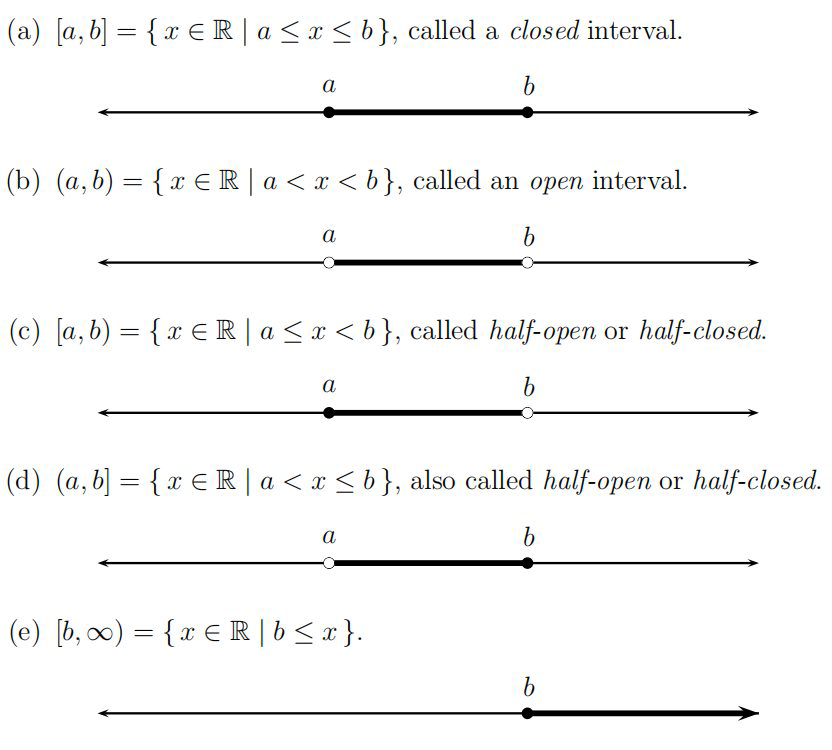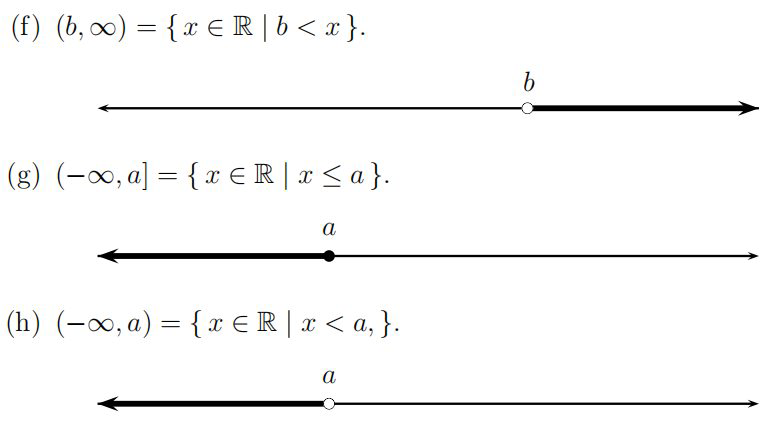It is also common to write $\mathbb{R}=(-\infty, \infty),$ the interval that covers the entire real line.

## Coordinate systems

### Cartesian plane

The Cartesian plane or xy-plane consists of all ordered pairs (x, y) as x and y range over all reals numbers, denoted by

$\mathbb{R}^{2}=\{(x, y) | x, y \in \mathbb{R}\},$

containing a horizontal $x$-axis $\{(x, 0) | x \in \mathbb{R}\}$ and a vertical $y-$axis $\{(0, y) | y \in \mathbb{R}\} .$ The axes are perpendicular and intersect at the origin $O=O(0,0) .$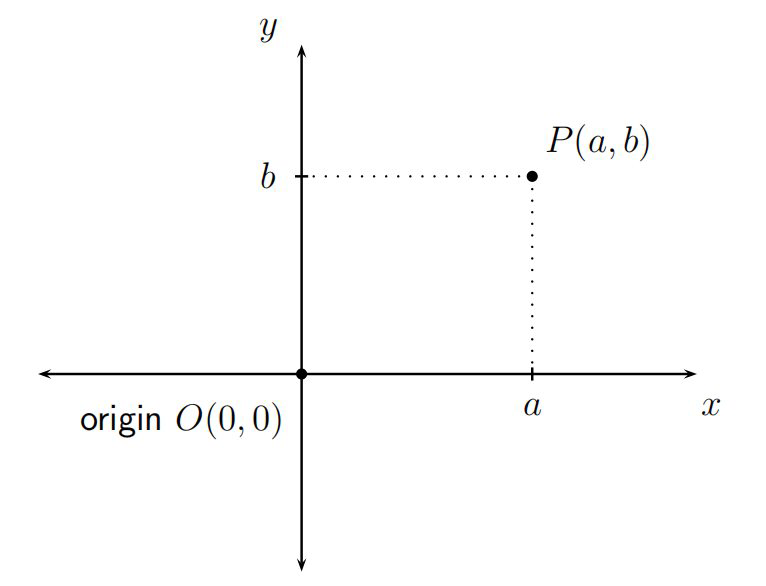If P = P(a, b) is a point in the xy-plane, then we call a the x-coordinate and b the y-coordinate of P, obtained by projecting P to the closest points on the x-axis and y-axis respectively. Together, we call the pair (a, b) the Cartesian coordinates of P. In the above diagram, the coordinates of P are positive. In general the coordinates can be positive, negative, zero or any mixture of these.

### Quadrants of the Cartesian plane

The Cartesian plane is divided by the coordinate axes into four quadrants.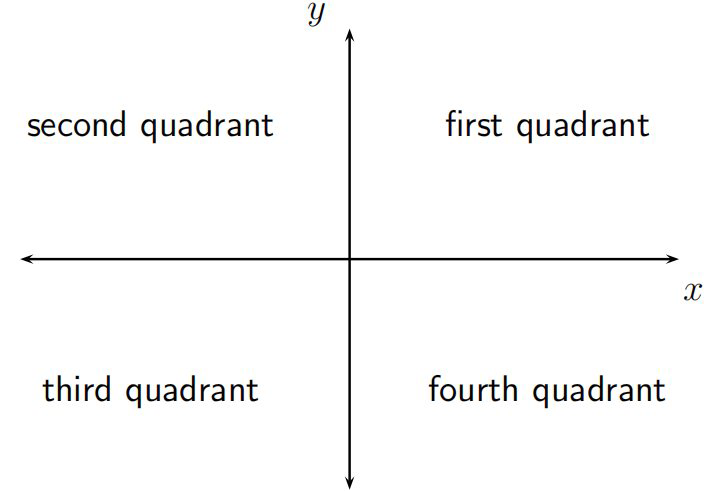The first quadrant consists of points with $(+,+)$ coordinates; the second with $(-,+)$ coordinates; the third with $(-,-)$ coordinates; the fourth with $(+,-)$ coordinates.

### Distance between two points in the Cartesian plane

The distance $d$ between points $P\left(x_{1}, y_{1}\right)$ and $Q\left(x_{2}, y_{2}\right)$ in the plane is given by the formula

$d=\sqrt{\left(x_{2}-x_{1}\right)^{2}+\left(y_{2}-y_{1}\right)^{2}}$

that is, the square root of the sum of the squares of the differences in their coordinates. This follows from the Theorem of Pythagoras, by creating a right-angled triangle with shorter sides parallel to the $x$ and $y$-axes.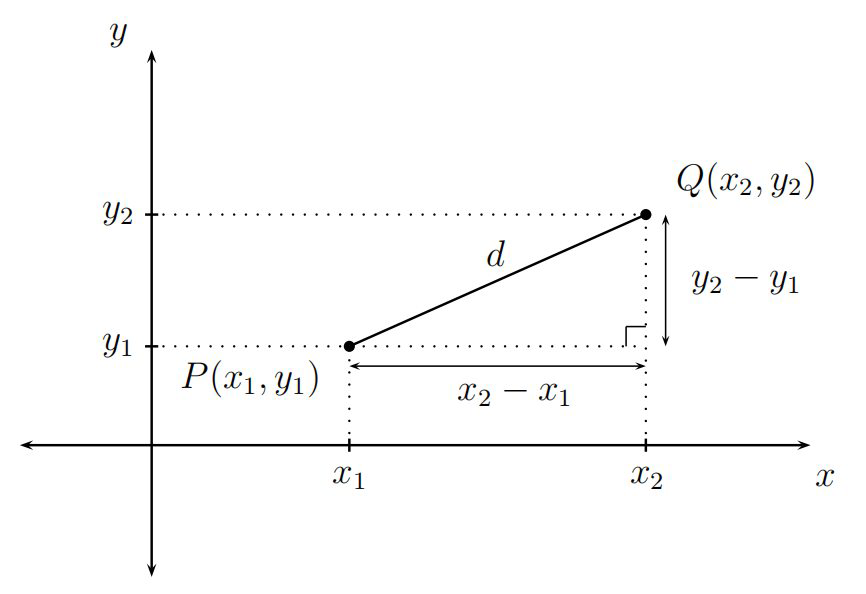In this diagram, the horizontal side of the triangle has length $x_{2}-x_{1}$ and the vertical side has length $y_{2}-y_{1} .$ The length of the hypotenuse is the distance $d$ between $P$ and $Q,$ so that $d^{2}=\left(x_{2}-x_{1}\right)^{2}+\left(y_{2}-y_{1}\right)^{2},$ by Pythagoras, and the above formula is immediate by taking positive square roots.

Note that the above diagram is particular in that it assumes the differences in coordinates are both positive. However, the mathematics works and the formula holds in all cases, including when these differences are zero or negative. This is because taking the square of a difference of two real numbers, inside the square root sign, produces the same outcome, regardless of the order in which the difference is taken.

### Coordinates in space

To model positions in space mathematically, we think of the xy-plane now as ‘horizontal’ (including the y-axis, which we previously thought of as being
‘vertical’), and introduce a third z-axis, another copy of the real line, which we think of as the new ‘vertical’, which is perpendicular to the xy-plane. All axes pass through a common point, again called the origin, and denoted by O = O(0, 0, 0). Every point P in space has coordinates (a, b, c), for some real numbers a, b and c, which are formed by projecting P to the closest points on the x, y and z-axes respectively. In this way we identify space with the set of triples of real numbers, denoted by

$\mathbb{R}^{3}=\{(a, b, c) | a, b, c \in \mathbb{R}\}$

The $x$-axis is identified with the subset of triples $\{(x, 0,0) | x \in \mathbb{R}\},$ the $y$-axis with the subset $\{(0, y, 0) | y \in \mathbb{R}\}$ and the $z$-axis with the subset $\{(0,0, z) | z \in \mathbb{R}\} .$ In the following diagram, only the positive parts of the axes emanating out of the origin are drawn, and you have to imagine the negative halves of the axes extending behind the page (for the $x$-axis), to the far left (for the $y$-axis) and far below (for the $z$-axis).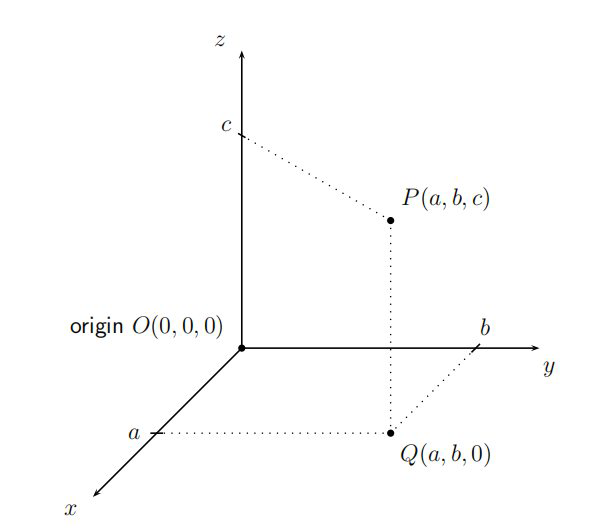Imagine the point P hovering in space above the (horizontal) xy-plane. We project down (vertically) onto the xy-plane to get to some point Q, say, which has projections a and b on the x and y-axes respectively. The closest point on the z-axis to Q is the origin, with z-value 0, so the coordinates of Q become (a, b, 0). If we move from P directly across (horizontally) to the z-axis then we meet it at some point, say c. Then the triple of real numbers (a, b, c) that we get in this way become the coordinates of P.

### Distance between two points in space

The formula for the distance between two points in
space generalises the earlier formula for the distance between two points in the xy-plane. As a special case consider the distance from P(a, b, c) to the origin O(0, 0, 0).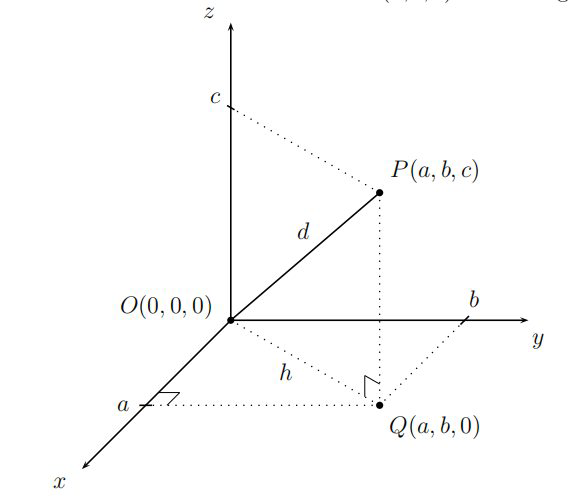Add a line segment joining O to P, whose length is the distance d from P to O. The points O, P and Q form a right-angled triangle with shorter side-lengths c (the closest point to P on the z-axis) and the distance from O to Q, which we denote by h.

By Pythagoras, $d^{2}=h^{2}+c^{2} .$ But, by Pythagoras again, $h^{2}=a^{2}+b^{2},$ since $h$ is the length of the hypotenuse formed by the right-angled triangle using $O, Q$ and the point on the $x$-axis labelled by $a$ , whose shorter side-lengths are now just $a$ and $b$ . Hence

$d^{2}=h^{2}+c^{2}=a^{2}+b^{2}+c^{2}$

Taking the square root, we deduce that the distance from $P(a, b, c)$ to the origin $O(0,0,0)$ is

$d=\sqrt{a^{2}+b^{2}+c^{2}}$

This diagram assumed $a, b$ and $c$ are positive, but the mathematics works regardless of the signs of the coordinates of $P .$
More generally, the distance $d$ between points $P\left(x_{1}, y_{1}, z_{1}\right)$ and $Q\left(x_{1}, y_{2}, z_{2}\right)$ is given by the formula

$d=\sqrt{\left(x_{2}-x_{1}\right)^{2}+\left(y_{2}-y_{1}\right)^{2}+\left(z_{2}-z_{1}\right)^{2}}$

that is, the square root of the sum of the squares of the differences in their coordinates. This can be shown using a similar but more elaborate diagram to the above, or alternatively can be deduced from the special case, by translating both of the points parallel to themselves so that one of them goes to the origin. If $P$ is translated to $O(0,0,0)$ then $Q$ is translated to the point with coordinates $\left(x_{2}-x_{1}, y_{2}-y_{1}, z_{2}-z_{1}\right),$ and the general result now follows from the special case.

### The midpoint between two points in the plane or in space

The midpoint of the line segment joining points P(x1, y1, z1) to Q(x2, y2, z2) in space has coordinates

$\left(\frac{x_{1}+x_{2}}{2}, \frac{y_{1}+y_{2}}{2}, \frac{z_{1}+z_{2}}{2}\right)$

that is, the triple formed by taking the averages of the respective coordinates of $P$ and Q. By ignoring the $z$-coordinate in the previous formula, we see that the midpoint of the line segment joining points $P\left(x_{1}, y_{1}\right)$ and $Q\left(x_{2}, y_{2}\right)$ in the plane has coordinates

$\left(\frac{x_{1}+x_{2}}{2}, \frac{y_{1}+y_{2}}{2}\right)$

## Distance and absolute value

### Absolute value

If $\alpha \in \mathbb{R}$ then the absolute value or magnitude of $\alpha,$ denoted by $|\alpha|,$ is the distance from $\alpha$ to 0, regarded as points on the real number line, so that

|\alpha|=\left\{\begin{aligned} \alpha & \text { if } \alpha \geq 0 \\-\alpha & \text { if } \alpha<0 \end{aligned}\right.

Note that $|\alpha|$ just reproduces itself if $\alpha$ is positive or zero, but takes its negative (which turns out to be positive) if $\alpha$ is negative. For example, $|2|=2$ and

$|-2|=-(-2)=2$

The absolute value is always nonnegative.

### Useful laws, including the triangle inequality

The following laws hold, for all $\alpha, \beta, \gamma \in \mathbb{R},$ with $\gamma \neq 0$ :

$|-\alpha|=|\alpha|=\sqrt{\alpha^{2}}, \quad|\alpha \beta|=|\alpha| \beta|, \quad| \alpha / \gamma|=| \alpha|/| \gamma|, \quad| \alpha+\beta| \leq| \alpha|+| \beta |,$

the last of which is known as the triangle inequality. For example,

$|2|=|-2|=\sqrt{2^{2}}=\sqrt{(-2)^{2}}=\sqrt{4},$

$|2||-3|=|2 \times(-3)|=|-6|=6 \quad \text { and } \quad \frac{|2|}{|-3|}=\left|\frac{2}{-3}\right|=\frac{2}{3}.$

Observe further that

$|2+(-3)|=|-1|=1<5=2+3=|2|+|-3|,$

consistent with the triangle inequality (using “less than” in this particular case, which is included in “less than or equals”). The reason for the terminology “triangle inequality” may seem cryptic, but is related to the fact that the shortest distance between any two points P and Q in the plane or in space is along the straight line joining them. One may think of this straight line as one edge of a triangle made with P and Q and a third point R typically not on this shortest straight line segment. In our context with absolute values, all points are constrained to lie in the real line, so the “triangles” PQR that arise turn out to be degenerate (having no area).

### Distance between two points in the real line

Distance between two points in the real line: If $\alpha, \beta \in \mathbb{R}$ then $|\alpha-\beta|$ is the distance between $\alpha$ and $\beta,$ as points on the real number line.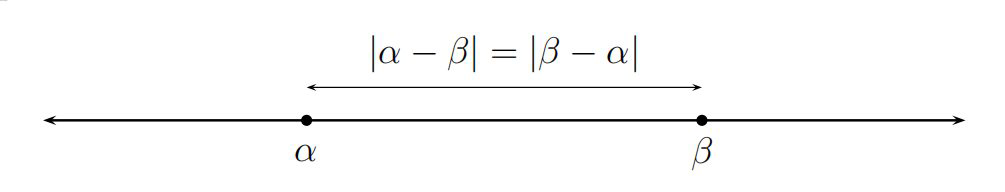Note that the distance between $\alpha$ and $-\beta,$ or between $-\alpha$ and $\beta$ is

$|\alpha+\beta|=|\alpha-(-\beta)|=|\beta-(-\alpha)|$

## Lines and circles in the plane

### Lines in the plane

$A$ line in the $x y$ -plane is the set of points $(x, y)$ satisfying an equation
either of the form $y=m x+k$ (the nonvertical case), where $m$ is the slope and $k$ is the $y$-intercept, or $x=\ell$ (the vertical case), where $\ell$ is the $x$-intercept. All lines may be put in the form

$a x+b y=c$

for some constants a, b and c.

This equation is only interesting by assuming that at least one of $a$ or $b$ is nonzero. If $a=0$ then this assumption forces $b$ to be nonzero, in which case the equation describes a horizontal line $\left(y=\frac{c}{b}\right) .$ If $b=0$ then this assumption forces $a$ to be nonzero, in which case the equation describes a vertical line $\left(x=\frac{c}{a}\right) .$

### Slope of a line determined by two distinct points

A line is completely determined by two distinct points. Let P(x1, y1), Q(x2, y2) be distinct points in the plane. The slope of the line joining P to Q is

$\frac{\text { vertical rise }}{\text { horizontal run }}=\frac{y_{2}-y_{1}}{x_{2}-x_{1}}=\frac{y_{1}-y_{2}}{x_{1}-x_{2}}$

if $x_{1} \neq x_{2},$ and infinite if $x_{1}=x_{2}$ (when the line is vertical). An equation of the line through $P$ and $Q$ is

$y-y_{1}=\left(\frac{y_{2}-y_{1}}{x_{2}-x_{1}}\right)\left(x-x_{1}\right)$

provided that $x_{1} \neq x_{2}$.

If the slope is positive then the line slopes upwards as one moves from left to right. If the slope is negative then the line slopes downwards as one moves from left to right. If the slope is zero then the line is horizontal.
If the slope is m, then one moves m units vertically (upwards if m is positive and downwards if m is negative) for each unit that one moves horizontally towards the right.

### Parallel and perpendicular lines

Two nnonvertical lines in the $x y$ -plane with slopes $m_{1}$ and $m_{2}$ respectively are parallel if $m_{1}=m_{2},$ and perpendicular if $m_{1} m_{2}=-1,$ in which case $m_{1}$ and $m_{2}$ become negative reciprocals of each other.

### Equation of a circle

A circle in the $x y$ -plane, centred at $P\left(x_{0}, y_{0}\right)$ with radius $r$ is the set of points $(x, y)$ satisfying the equation

$\left(x-x_{0}\right)^{2}+\left(y-y_{0}\right)^{2}=r^{2} \text { . }$

Any tangent to the circle is perpendicular to the radius joining the centre of the circle to the intersection point.

## Vocabulary

fraction：分数
rationals：有理
irrationals：无理
significant figures：有效数字
scientific notation：科学计数法
subtraction：减法
surd：无理数,不尽根数
surd expression：不尽根式